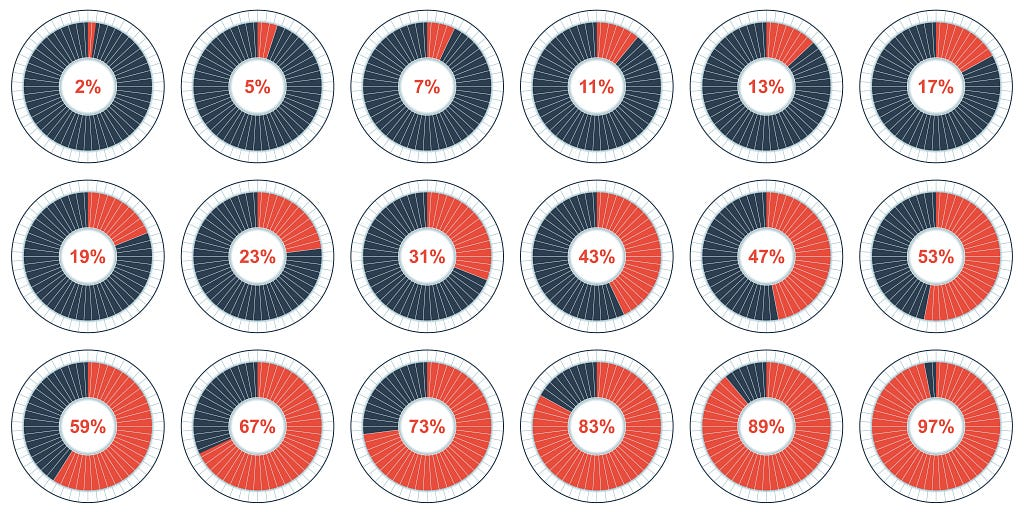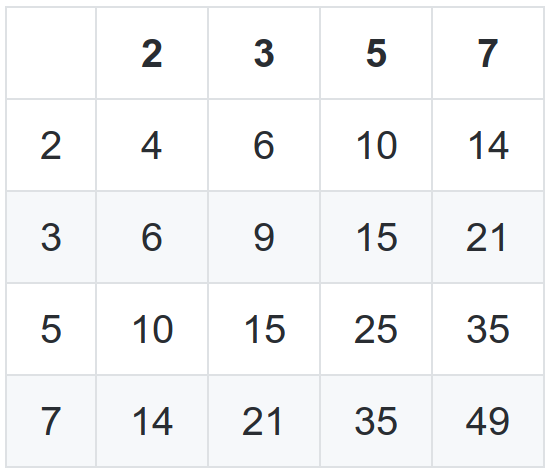DEV Community is a community of 788,395 amazing developers

We're a place where coders share, stay up-to-date and grow their careers.

Nicolas Sebastian Vidal

Posted on • Originally published at Medium on

The Sieve of Eratosthenes

Generating a multiplication table with the first N prime numbers.Introduction

This post is for walking you through the steps I have taken for creating a command line tool for printing out a multiplication table of the first N prime numbers. The result is a gem called primes_table and for the related code, you can take a look to this repository.

Stack:

• Methadone an awesome project for creating command-line tools;
• Ruby version 2.5.0;
• RSpec as a testing framework;
• Simplecov for code coverage;
• CodeClimate for engineering process insights and automated code review;
• SemaphoreCI for continuous integration;

Input

The command line tool will receive two parameters rows and columns.

Specifications:

• By default the table will be generated as a matrix of 10X10;
• Only values greater or equal than 10 will be considered for specifying rows or columns;
• If you enter a value minor than 10, it will default to 10;
• Only integer values are considered. For example, if you enter a string, it will be converted to an integer, the result will be 0 (zero) and will default to 10 because zero is minor than 10;

Output

A multiplication table with the first N prime numbers. The first row and column are the respective prime numbers.

For example, the default output will have as the first column, the first prime numbers until number 10. This means numbers 2, 3, 5 and 7. The same with the first row. The following image should help you visualize the desired output:Creating the gem

With methadone installed you just need to run:

That command will generate the skeleton of your command line tool.

I have created an extra couple of options to add to the ones already included when you create the project. After that, all the options are:

• -h, --help to see the available options;
• -r, --rows ROWS to specify how many rows;
• -c, --columns COLUMNS to specify how many columns;
• --version it will give you the version of the gem you are using;

The following code handles the case where no options are entered. The default option is always going to be 10 for rows and columns.

Naive approach

The first approach was to loop over each number from 2 to N. This means that per each number I was iterating over it in order to check whether there was not another divisor than himself.

On the other hand, we have the Matrix class with a private method called header that returns the list of primes numbers I want, until the given N.

Making improvements

Once I got that working, I thought that it has to be a better approach to solving it. I'm iterating from 2 to N and over that list of numbers, I'm iterating again form 2 to N[i] checking for primality. So I checked in one of the books I have entitled Cracking the Coding Interview and there it was. Two improvements that can be done.

The first improvement was to iterate not from 2 to N[i] but to the square root of this number. So the code will look something like this:

The sqrt(n) is sufficient because for every number a which divides n evenly, there is a complement b , where a * b = n . If a > sqrt(n) , then b < sqrt(n) (since (sqrt(n))**2 = n). We, therefore, don't need to check n 's primality, since we would have already checked with b .

The next improvement is to implement The Sieve of Eratosthenes. This is a highly efficient way to generate a list of primes. It works by recognizing all non-prime numbers are divisible by a prime number.

We start with a list of all numbers up through some maximum value. First, we cross off all numbers divisible by 2. Then, look for the next prime (the next non-crossed off number) and cross off all numbers divisible by it. By crossing off all numbers divisible by 2, 3, 5, 7, 11, and so on, we wind up with a list of prime numbers from 2 through a maximum.

As you can see the method header calls process\_flags at the end. This is not part of The Sieve of Eratosthenes calculations since we are using it only to get the primes numbers based on the flags we have:

At the end our class Matrix will look something like this:

A couple of things to notice here is that I'm not using the class Prime anymore. I have taken this decision because the actions I want to perform are related to the Matrix itself and at the moment I don't really see the need for spreading the logic into another class. In the future, if the project grows in some way, I could evaluate how to scale it. But at the moment, I don't have enough information to do it.

Notes

As I mentioned at the beginning of this post, all code is Open Source and it can be found in this repository.

If you think that I should have taken a different approach or maybe you have a couple of ideas that you would like to implement, don’t doubt it and make a PR right away ☺.

One thing to notice is that right now I’m calculating the list of prime numbers for both, rows and columns. I think this could be improved. If rows are equal to columns it should be enough with calculating the list of primes only once.

Another improvement could be to only calculate the list of primes of the biggest number that was entered as row or column. In the process of calculating the list of prime numbers for the biggest number entered, we should be able to collect the prime numbers for the minor value as well.